Below are a few examples of using plot() function in R:

# Example 1: Basic Scatterplot in RA Scatterplot with Default Specifications in Base R

# Example 2: Scatterplot with User-Defined Title & Labels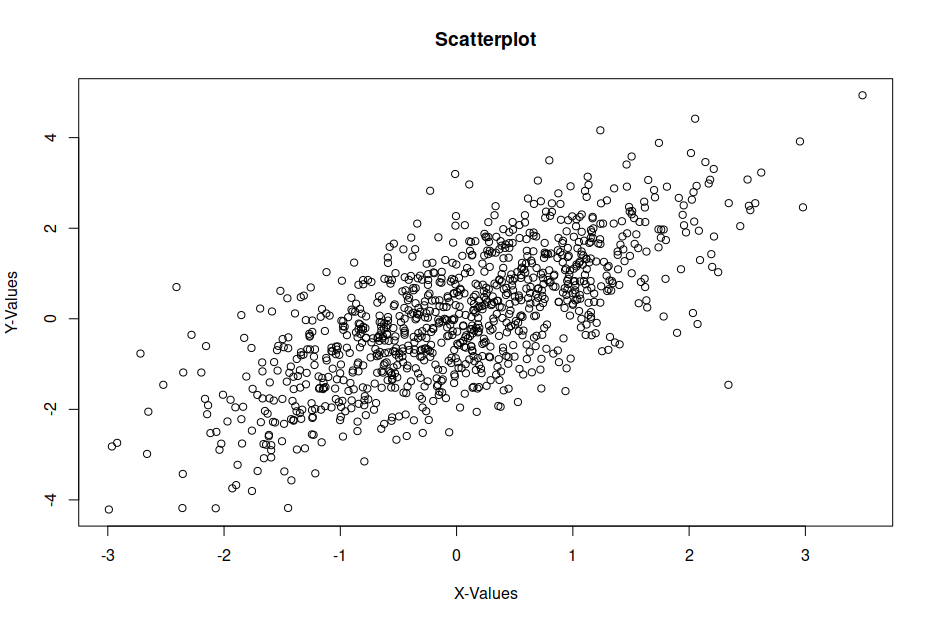Scatterplot with User-Defined Main Title & Axis Label

# Example 3: RegressionRegression line in R

# Example 4: Density Plot in R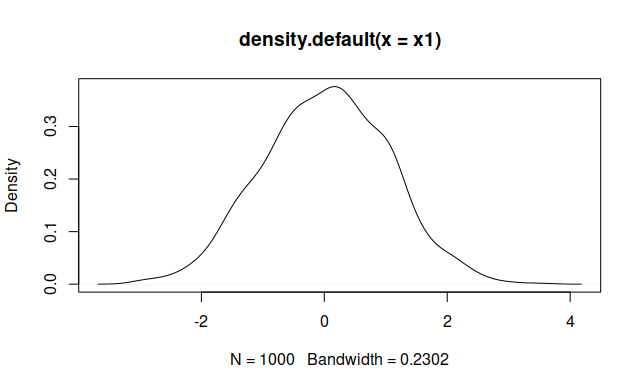Density plot in R

# Example 5: Plot with Colors & PCH According to Group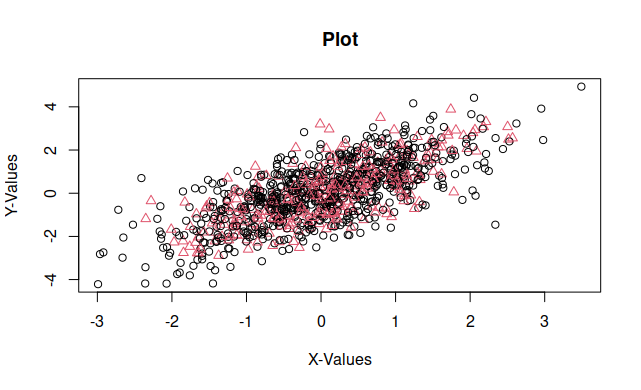# Example 6: Plot Multiple Densities in Same PlotPlot Multiple Densities in Same Plot

# Example 7: plot() and legend()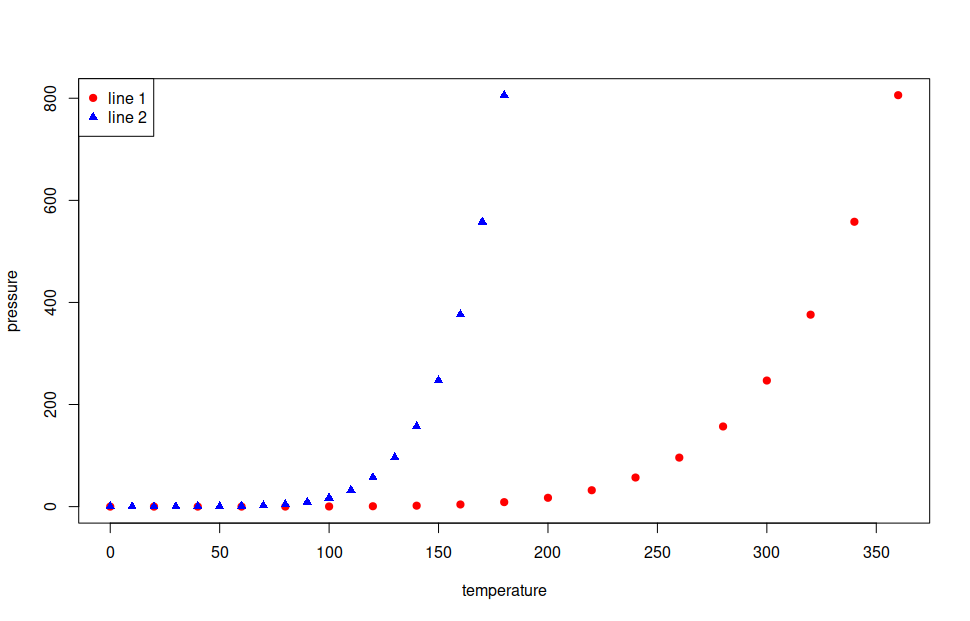plot() and legend()

# Example 8: Label data points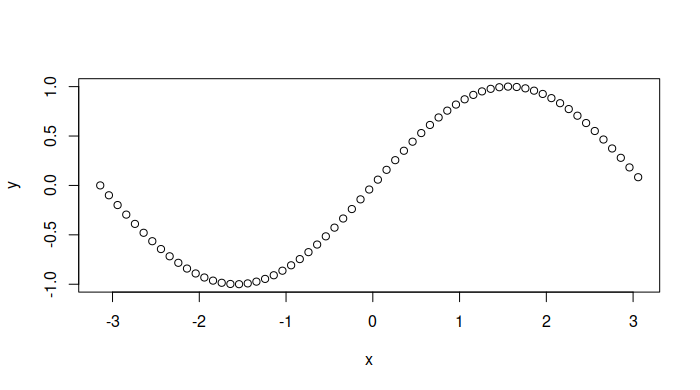Sine plot

# Example 9: Sine plot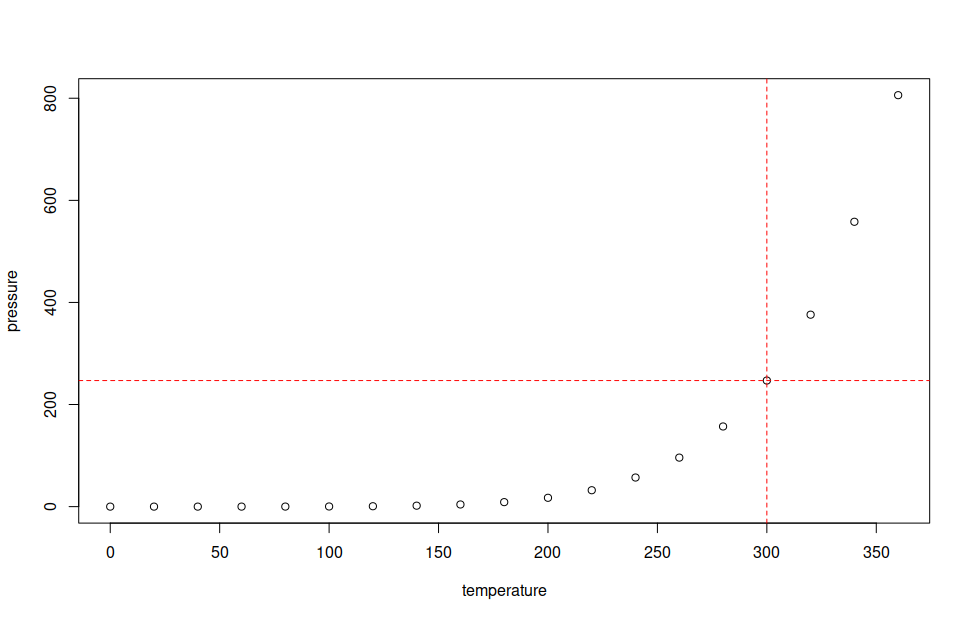Label data points

# Example 10: Matrix of Scatterplots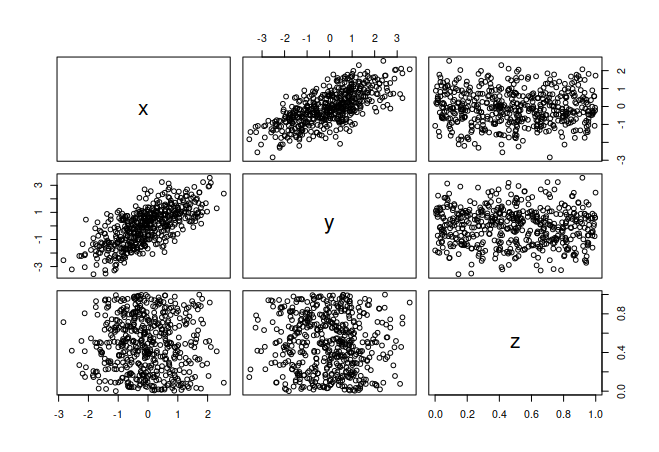Matrix Created with pairs()

My site is free of ads and trackers. Was this post helpful to you? Why not